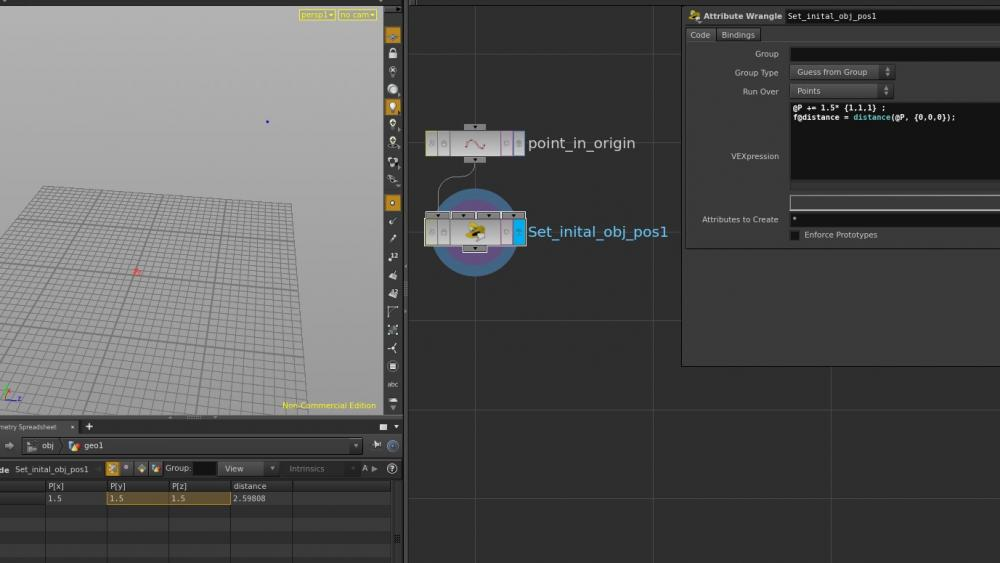# VEX - moving obj in a certain direction by a certain amount

## Recommended Posts

Hi

I have an object and I want to move it lets say 1.5 units in a certain direction ( so move it along a certain vector ), so I tried what you can see in the picture.

Of course this doesn't work, because 1.5*{1,1,1} will result in moving the obj of 1.5 units in every axis.

So how can I work out the correct numbers to move the obj by a certain amount in a certain direction?##### Share on other sites

You need to normalize your direction vector first before the multiplication.

```vector dir = {1, 1, 1};
v@P += 1.5 * normalize(dir);```

•1

##### Share on other sites
```float distance = chf("distance");
vector direction = chv("direction");
@P += normalize(direction) * distance;```

##### Share on other sites
Quote

You need to normalize your direction vector first before the multiplication.

Ok Thanks it works!

As far as I knew normalizing something would have made it equal to 1, could you explain better what it does?

##### Share on other sites

after normalizing the vector (dividing each component by its length) it is exactly one unit long.

## Create an account

Register a new account